# NCERT Solutions for Class 9 Maths Chapter 7 Exercise 7.4 – Triangles

Download NCERT Solutions for Class 9 Maths Chapter 7 Exercise 7.4 – Triangles. This Exercise contains 6 questions, for which detailed answers have been provided in this note. In case you are looking at studying the remaining Exercise for Class 9 for Maths NCERT solutions for Chapter 8 or other Chapters, you can click the link at the end of this Note.

### NCERT Solutions for Class 9 Maths Chapter 7 Exercise 7.4 – Triangles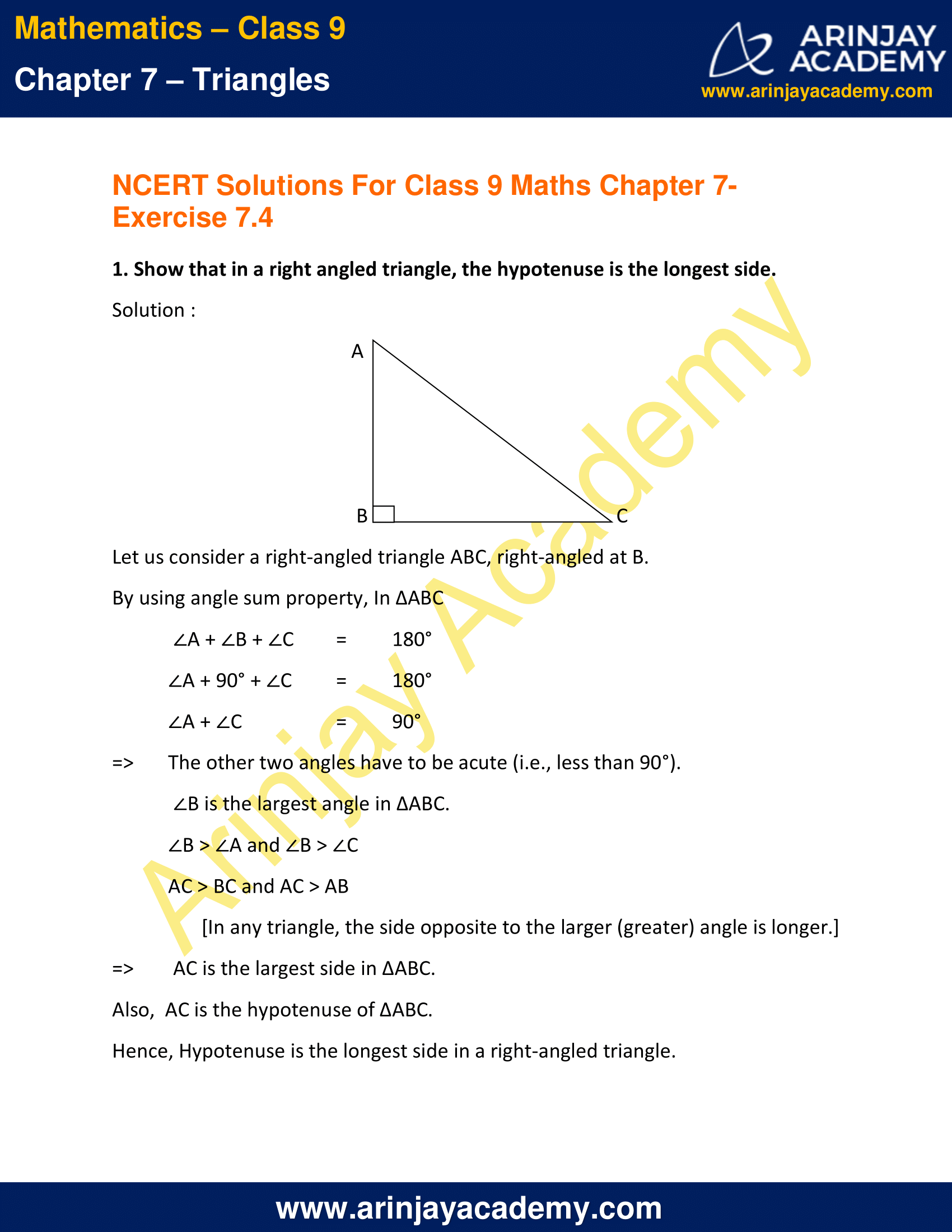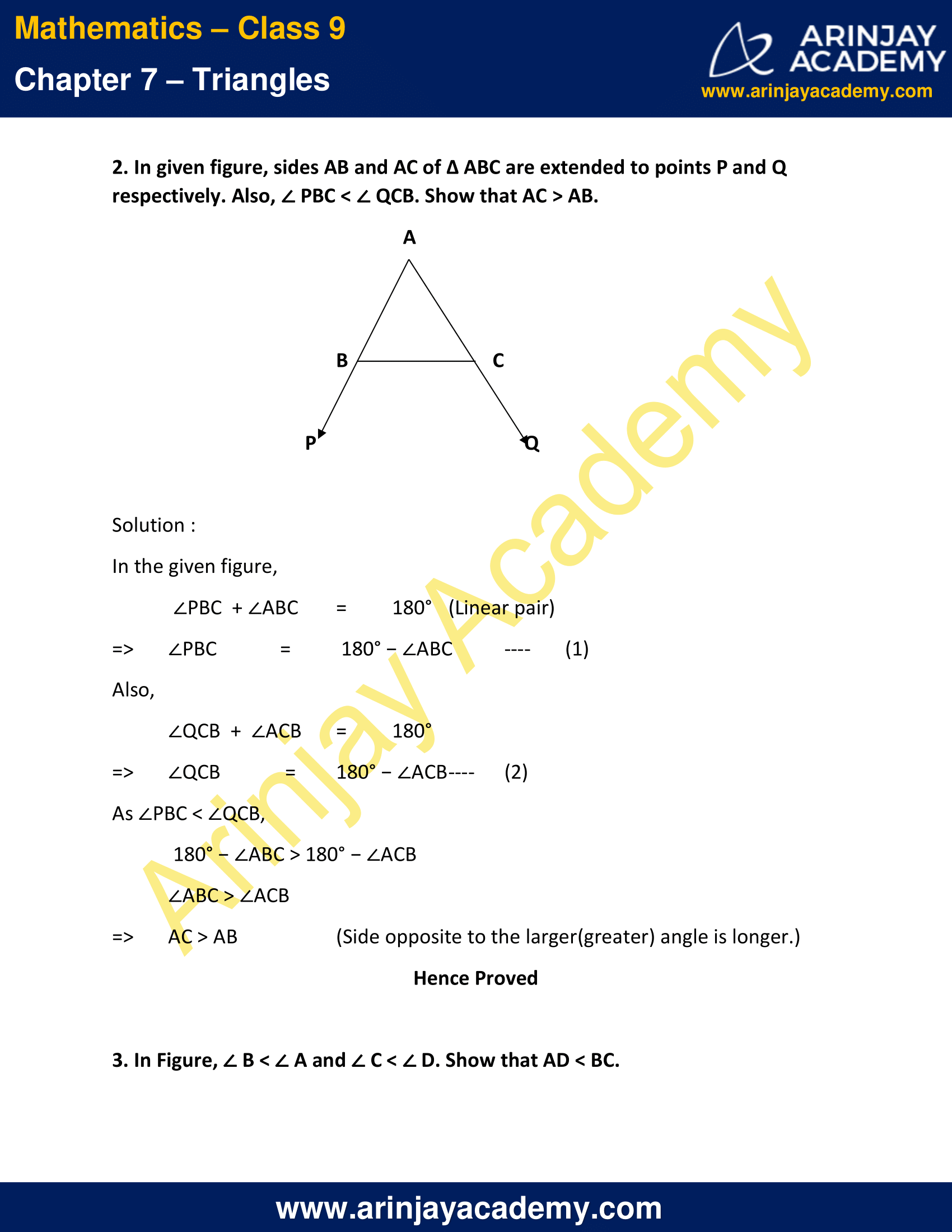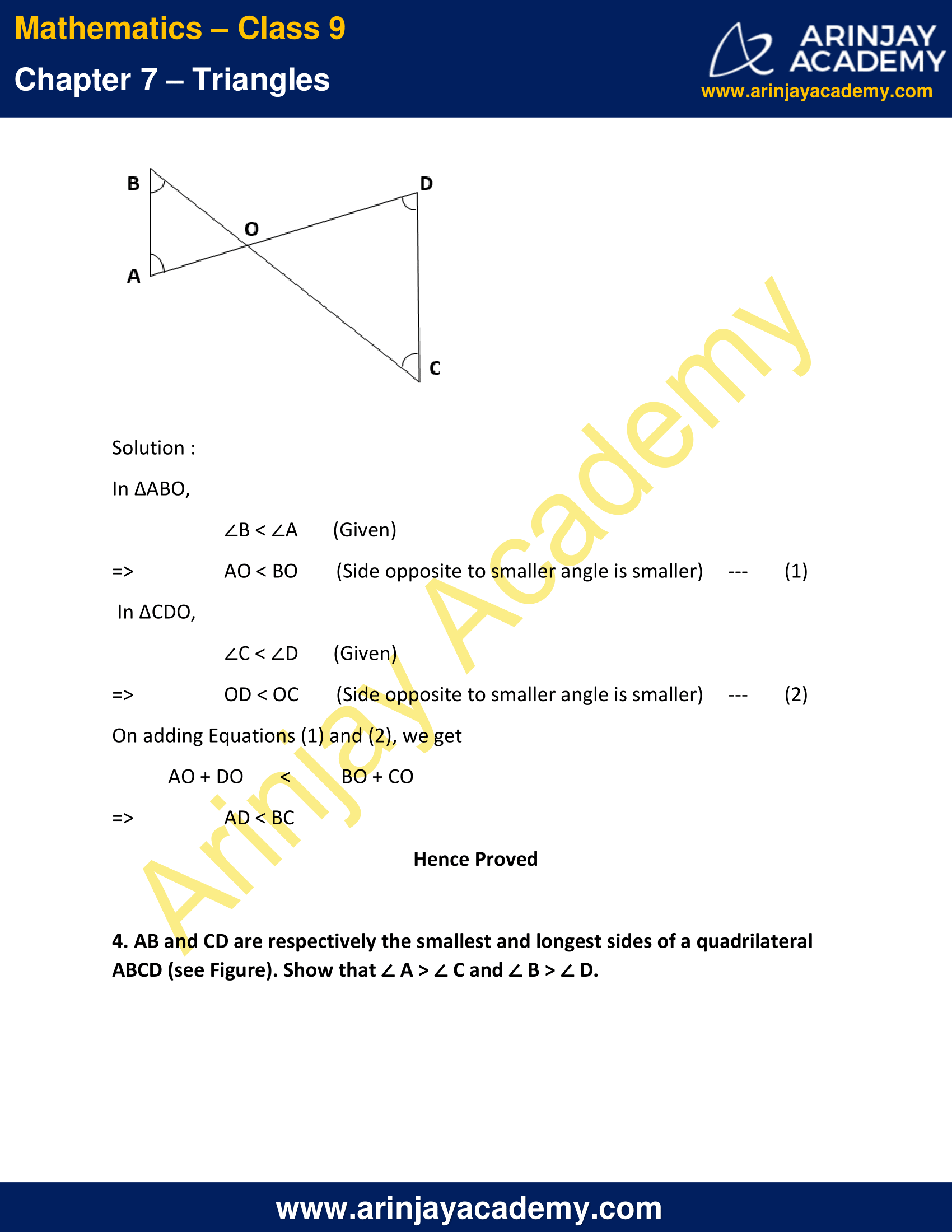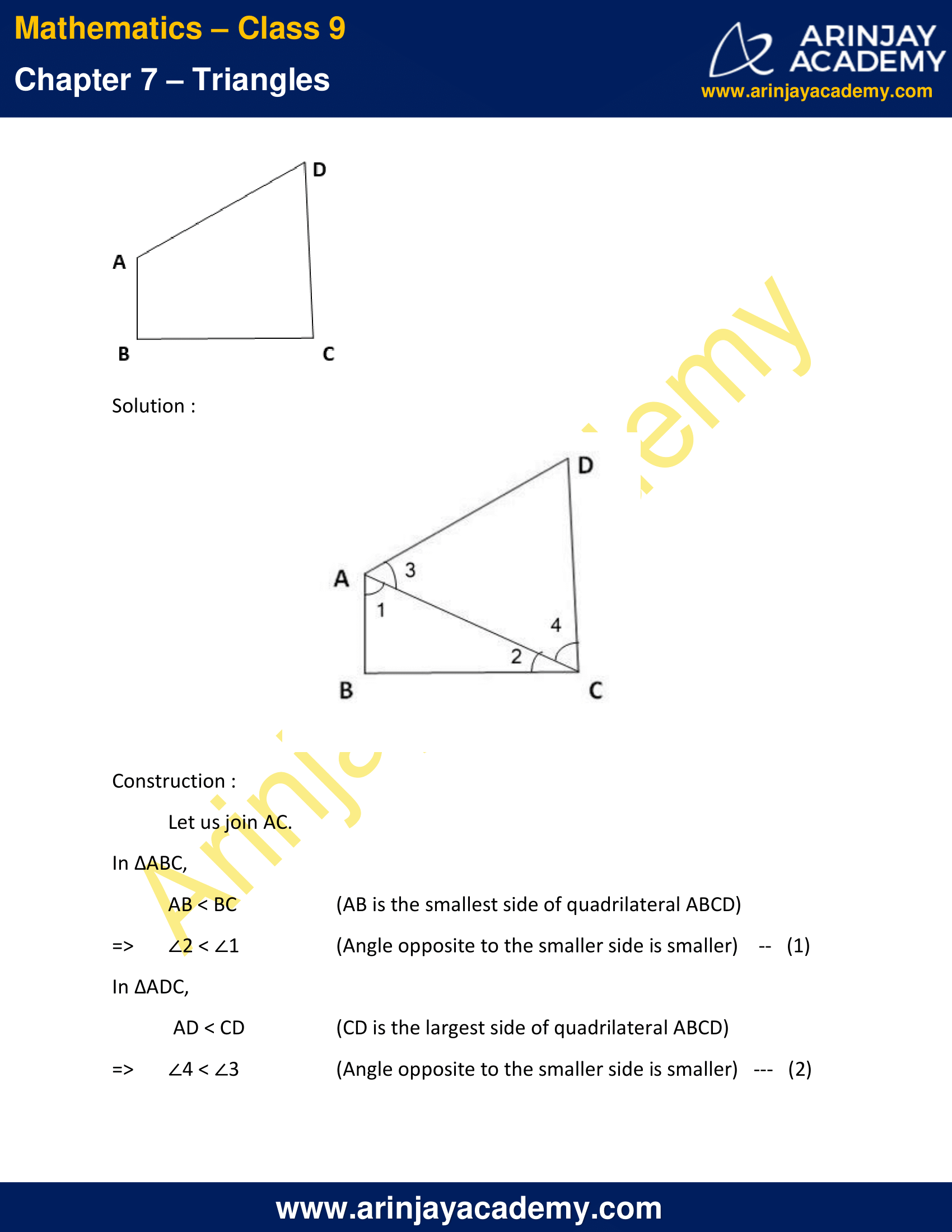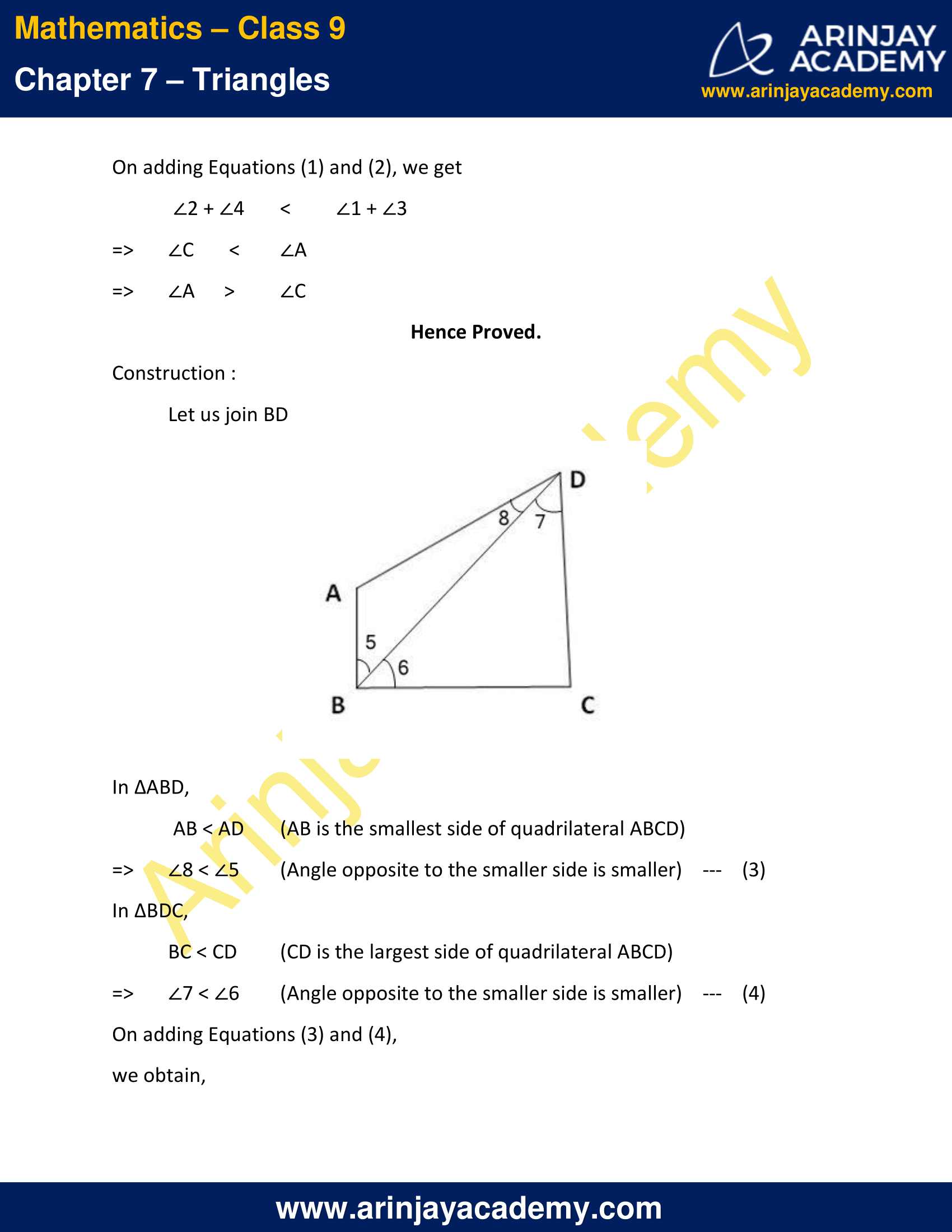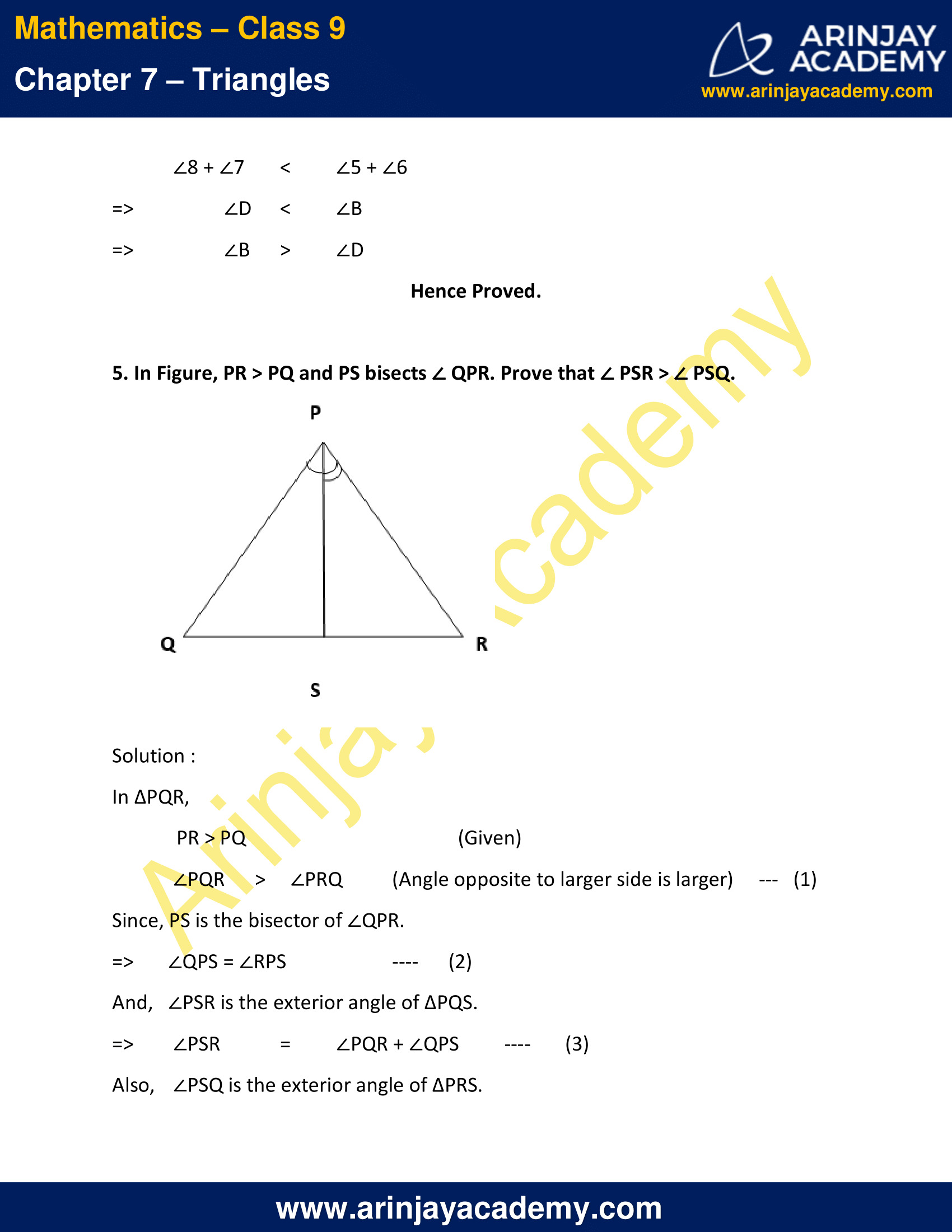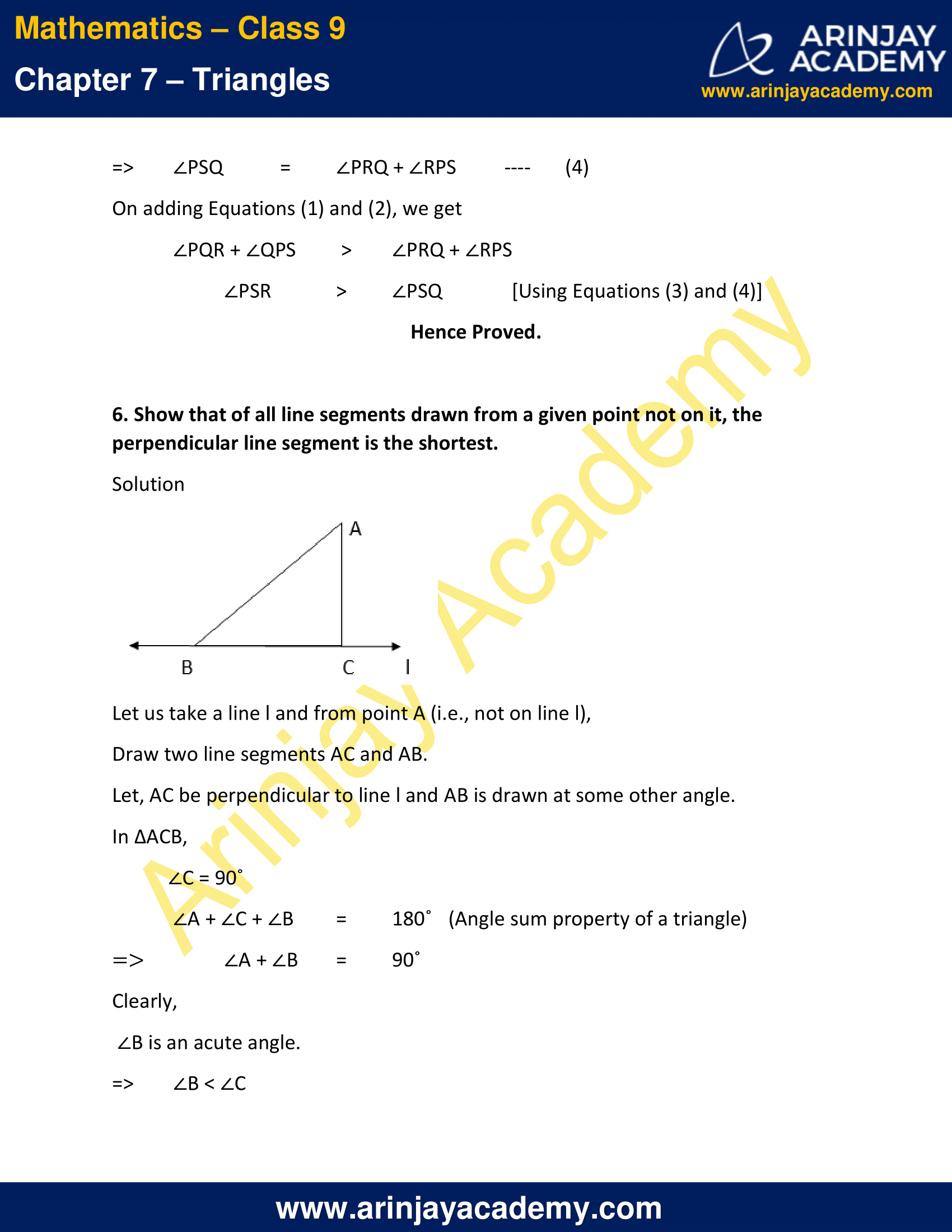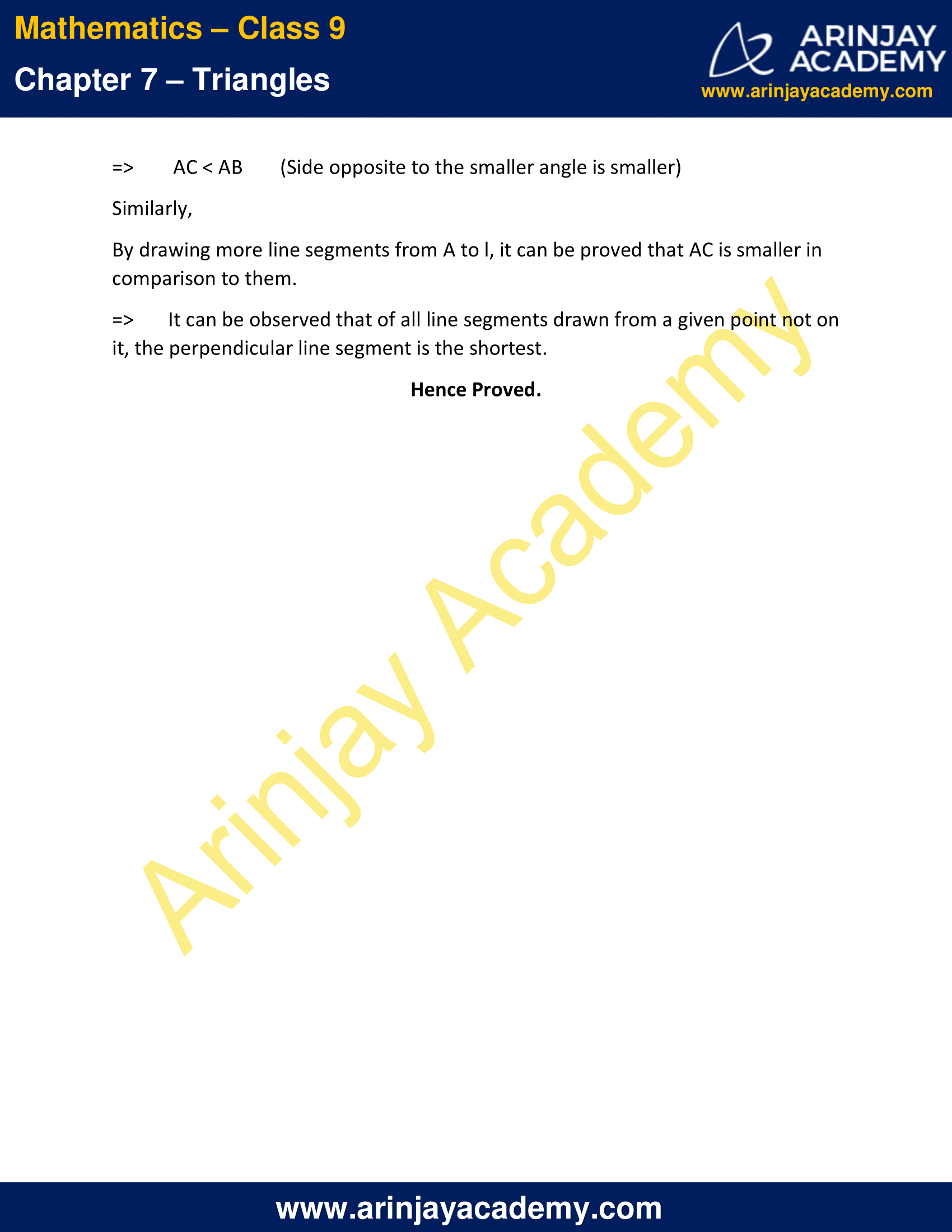NCERT Solutions for Class 9 Maths Chapter 7 Exercise 7.4 – Triangles

1. Show that in a right angled triangle, the hypotenuse is the longest side.

Solution :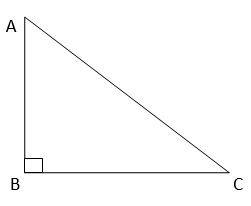Let us consider a right-angled triangle ABC, right-angled at B.
By using angle sum property, In ∆ABC
∠A + ∠B + ∠C = 180°
∠A + 90° + ∠C = 180°
∠A + ∠C = 90°
=> The other two angles have to be acute (i.e., less than 90º).
∠B is the largest angle in ∆ABC.
∠B > ∠A and ∠B > ∠C
AC > BC and AC > AB
[In any triangle, the side opposite to the larger (greater) angle is longer.]
=> AC is the largest side in ∆ABC.
Also, AC is the hypotenuse of ∆ABC.
Hence, Hypotenuse is the longest side in a right-angled triangle.

2. In given figure, sides AB and AC of ∆ ABC are extended to points P and Q respectively. Also, ∠ PBC < ∠ QCB. Show that AC > AB.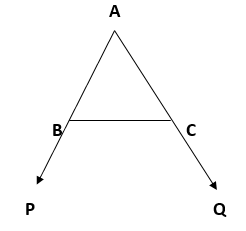Solution :
In the given figure,
∠PBC + ∠ABC = 180° (Linear pair)
=> ∠PBC = 180° − ∠ABC —- (1)
Also,
∠QCB + ∠ACB = 180°
=> ∠QCB = 180° − ∠ACB —- (2)
As ∠PBC < ∠QCB, 180° − ∠ABC > 180° − ∠ACB
∠ABC > ∠ACB
=> AC > AB (Side opposite to the larger(greater) angle is longer.)
Hence Proved

3. In Figure, ∠ B < ∠ A and ∠ C < ∠ D. Show that AD < BC.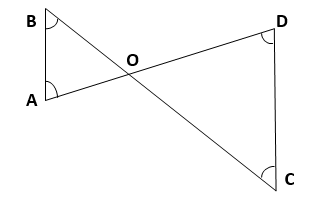Solution :
In ∆ABO,
∠B < ∠A (Given) => AO < BO (Side opposite to smaller angle is smaller) — (1)
In ∆CDO,
∠C < ∠D (Given) => OD < OC (Side opposite to smaller angle is smaller) — (2)
On adding Equations (1) and (2), we get
AO + DO < BO + CO => AD < BC
Hence Proved

4. AB and CD are respectively the smallest and longest sides of a quadrilateral ABCD (see Figure). Show that ∠ A > ∠ C and ∠ B > ∠ D.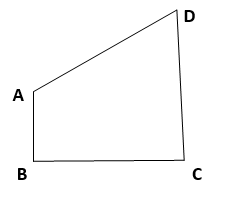Solution :

Construction :
Let us join AC.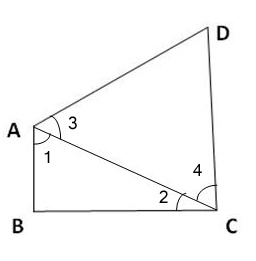In ∆ABC,
AB < BC (AB is the smallest side of quadrilateral ABCD)
=> ∠2 < ∠1 (Angle opposite to the smaller side is smaller) — (1)
In ∆ADC,
AD < CD (CD is the largest side of quadrilateral ABCD)
=> ∠4 < ∠3 (Angle opposite to the smaller side is smaller) — (2)
On adding Equations (1) and (2), we get
∠2 + ∠4 < ∠1 + ∠3
=> ∠C < ∠A
=> ∠A > ∠C
Hence Proved.

Construction :
Let us join BD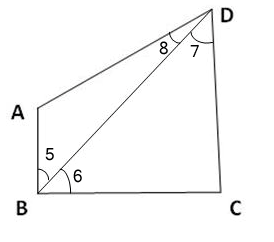In ∆ABD,
AB < AD (AB is the smallest side of quadrilateral ABCD)
=> ∠8 < ∠5 (Angle opposite to the smaller side is smaller) — (3)
In ∆BDC,
BC < CD (CD is the largest side of quadrilateral ABCD)
=> ∠7 < ∠6 (Angle opposite to the smaller side is smaller) — (4)
On adding Equations (3) and (4),
we obtain,
∠8 + ∠7 < ∠5 + ∠6 => ∠D < ∠B => ∠B > ∠D
Hence Proved.

5. In Figure, PR > PQ and PS bisects ∠ QPR. Prove that ∠ PSR > ∠ PSQ.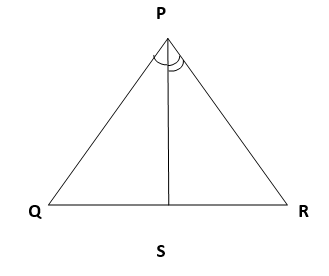Solution :
In ∆PQR,
PR > PQ (Given)
∠PQR > ∠PRQ (Angle opposite to larger side is larger) — (1)
Since, PS is the bisector of ∠QPR.
=> ∠QPS = ∠RPS —- (2)
And, ∠PSR is the exterior angle of ∆PQS.
=> ∠PSR = ∠PQR + ∠QPS —- (3)
Also, ∠PSQ is the exterior angle of ∆PRS.
=> ∠PSQ = ∠PRQ + ∠RPS —- (4)
On adding Equations (1) and (2), we get
∠PQR + ∠QPS > ∠PRQ + ∠RPS
∠PSR > ∠PSQ [Using Equations (3) and (4)]
Hence Proved.

6. Show that of all line segments drawn from a given point not on it, the perpendicular line segment is the shortest.

Solution :Let us take a line l and from point A (i.e., not on line l),
Draw two line segments AC and AB.
Let, AC be perpendicular to line l and AB is drawn at some other angle.
In ∆ACB,
∠C = 90˚
∠A + ∠C + ∠B = 180˚ (Angle sum property of a triangle)
=> ∠A + ∠B = 90˚
Clearly,
∠B is an acute angle.
=> ∠B < ∠C => AC < AB (Side opposite to the smaller angle is smaller)

Similarly, By drawing more line segments from A to l, it can be proved that AC is smaller in comparison to them.

=> It can be observed that of all line segments drawn from a given point not on it, the perpendicular line segment is the shortest.
Hence Proved.

NCERT Solutions for Class 9 Maths Chapter 7 Exercise 7.4 – Triangles, has been designed by the NCERT to test the knowledge of the student on the following topics:-

• Inequalities in a Triangle
– If two sides of a triangle are unequal, the angle opposite to the longer side is larger (or greater).
– In any triangle, the side opposite to the larger (greater) angle is longer.
– The sum of any two sides of a triangle is greater than the third side.

Download NCERT Solutions for Class 9 Maths Chapter 7 Exercise 7.4 – Triangles